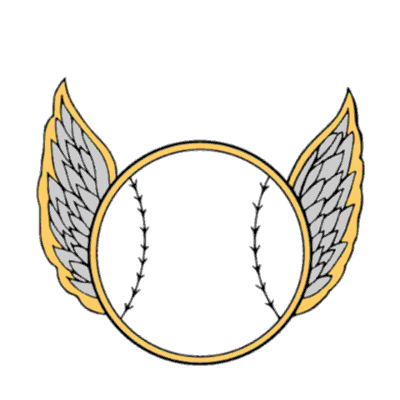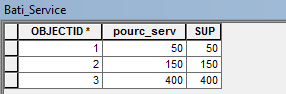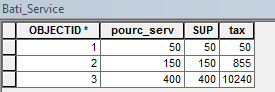# Problem in a Buton addin

496
5
09-21-2018 06:48 AMNew Contributor II

I have created a button and I want that when a click a field in the attribute table is filled after having made a calculation but it does not work.

This is my code :

class ButtonClass1(object):

def __init__(self):
self.enabled = True
self.checked = False
def onClick(self):
inTable = "Bati_Service"
fieldName = "tax"
expression = "getClass(float(tax))"
codeblock = def getClass(tax):
if (SUP < 100):
tax= (pourc_serv /100)* SUP * 2
elif (SUP > 100 And [SUP] < 200):
tax= (pourc_serv /100)* (SUP * 3.8 )
elif (SUP > 200 And < 400):
tax= (pourc_serv /100)* (SUP * 5.4 )
elif (SUP > 400):
tax= (pourc_serv /100)* (SUP * 6.4 )
# Execute CalculateField
arcpy.CalculateField_management("Bati_Service","tax","tax", "PYTHON","")

1 Solution

Accepted SolutionsbyMVP Regular Contributor

Here is the test code I was using inside ArcMap.  I've made a number of changes to your original code, including assuming the values for 'SUP' and 'pourc_serv' are in the 'Bati_Service' table; if not, the code will need to be adjusted on line 12.

``````import arcpy

# the table we are using
inTable = "Bati_Service"

# the field that will be created and updated
fieldName = "tax"

# expression is used to compute 'tax' field's value
# we need to pass 'SUP' and 'pourc_serv' to function --
#   code assumes these are fields in the table
expression = "getClass(float(!SUP!),float(!pourc_serv!))"

# this is the function that will compute the 'tax' value
codeblock ="""# code starts next line to make indentation easier to read
def getClass(SUP, pourc_serv):
if (SUP < 100.0):
return (pourc_serv /100.0)* SUP * 2.0
elif (SUP >= 100.0 and SUP < 200.0):
return (pourc_serv /100.0)* (SUP * 3.8 )
elif (SUP >= 200.0 and SUP < 400.0):
return (pourc_serv /100)* (SUP * 5.4 )
elif (SUP >= 400.0):
return (pourc_serv /100.0)* (SUP * 6.4 )"""

# if field exists a warning message may display, but the script should continue

# Execute CalculateField
# this will update the 'tax' field
arcpy.CalculateField_management(inTable,fieldName,expression, "PYTHON_9.3",codeblock)

print 'done'‍‍‍‍‍‍‍‍‍‍‍‍‍‍‍‍‍‍‍‍‍‍‍‍‍‍‍‍‍‍‍‍‍‍‍‍‍‍‍‍‍‍‍‍‍‍‍‍‍‍‍‍‍‍‍‍‍‍‍‍‍‍‍‍‍‍‍‍``````

This is what my 'Bati_Service' Table looked like, before:and after:If your table looks much different, add a photo showing some sample data.

If you get an error, please post it.  If the code works, then you can try pasting it into your button code.  The indentation should look similar to the example in my first posting.  Hope this helps.

5 RepliesbyMVP Honored Contributor

See the examples here for how to format calculate field codeblocks: Calculate Field—Help | ArcGIS for DesktopbyMVP Regular Contributor

Per Darren Wiens‌' suggestion, see the Calculate Field examples - particularly the one with the codeblock example with the 3 double quotes before and after the block since the one you want to use is over multiple lines.  You also need to use the expression to pass the SUP and pourc_serv variables (I assume these are fields in your table/feature).  The 'tax' will be the return value of the function.

And the CalculateField line also needs the proper items.  Here's my suggestions for your code. I have not tested it, however I would suggest testing lines 8-25 in its own script before putting it in a button add-in as the add-in is harder to debug.  If you get an error message, please post it so we can better help you.

``````class ButtonClass1(object):
def __init__(self):
self.enabled = True
self.checked = False

def onClick(self):
inTable = "Bati_Service"
fieldName = "tax"
expression = "getClass(float(!SUP!),float(!pourc_serv!))"
codeblock ="""
def getClass(SUP, pourc_serv):
if (SUP < 100.0):
return (pourc_serv /100.0)* (SUP * 2.0)
elif (SUP >= 100.0 and SUP < 200.0):
return (pourc_serv /100.0)* (SUP * 3.8 )
elif (SUP >= 200.0 and SUP < 400.0):
return (pourc_serv /100)* (SUP * 5.4 )
elif (SUP >= 400.0):
return (pourc_serv /100.0)* (SUP * 6.4 )"""

# Execute CalculateField
arcpy.CalculateField_management(inTable,fieldName,expression, "PYTHON_9.3",codeblock)‍‍‍‍‍‍‍‍‍‍‍‍‍‍‍‍‍‍‍‍‍‍‍‍‍‍‍‍‍‍‍‍‍‍‍‍‍‍‍‍‍‍‍‍‍‍‍‍‍‍‍‍‍‍‍‍‍‍‍‍‍‍‍‍‍‍‍‍‍‍‍‍‍‍‍‍‍‍‍‍‍‍‍‍‍‍‍‍‍‍‍‍‍‍‍‍‍‍‍‍‍‍‍‍‍‍‍‍‍‍‍‍‍‍‍‍‍‍‍‍‍‍‍‍‍``````New Contributor II

I tested the code in its own script before placing it in the button

complement but it did not work.

import arcpy

1. Set local variables

inTable="Bati_Service"

fieldName="tax"

expression="getClass(float(tax))"

codeblock="""def getClass(tax):

if (SUP :

Re: Problem in a Buton addin

Python - View the full discussionbyMVP Regular Contributor

Here is the test code I was using inside ArcMap.  I've made a number of changes to your original code, including assuming the values for 'SUP' and 'pourc_serv' are in the 'Bati_Service' table; if not, the code will need to be adjusted on line 12.

``````import arcpy

# the table we are using
inTable = "Bati_Service"

# the field that will be created and updated
fieldName = "tax"

# expression is used to compute 'tax' field's value
# we need to pass 'SUP' and 'pourc_serv' to function --
#   code assumes these are fields in the table
expression = "getClass(float(!SUP!),float(!pourc_serv!))"

# this is the function that will compute the 'tax' value
codeblock ="""# code starts next line to make indentation easier to read
def getClass(SUP, pourc_serv):
if (SUP < 100.0):
return (pourc_serv /100.0)* SUP * 2.0
elif (SUP >= 100.0 and SUP < 200.0):
return (pourc_serv /100.0)* (SUP * 3.8 )
elif (SUP >= 200.0 and SUP < 400.0):
return (pourc_serv /100)* (SUP * 5.4 )
elif (SUP >= 400.0):
return (pourc_serv /100.0)* (SUP * 6.4 )"""

# if field exists a warning message may display, but the script should continue

# Execute CalculateField
# this will update the 'tax' field
arcpy.CalculateField_management(inTable,fieldName,expression, "PYTHON_9.3",codeblock)

print 'done'‍‍‍‍‍‍‍‍‍‍‍‍‍‍‍‍‍‍‍‍‍‍‍‍‍‍‍‍‍‍‍‍‍‍‍‍‍‍‍‍‍‍‍‍‍‍‍‍‍‍‍‍‍‍‍‍‍‍‍‍‍‍‍‍‍‍‍‍``````

This is what my 'Bati_Service' Table looked like, before:and after:If your table looks much different, add a photo showing some sample data.

If you get an error, please post it.  If the code works, then you can try pasting it into your button code.  The indentation should look similar to the example in my first posting.  Hope this helps.New Contributor II

Thanks a lot Randy Burton the code works very well.

2018-09-25 4:52 GMT+02:00 Randy Burton <geonet@esri.com>: